# Calculus 1 : How to find increasing intervals by graphing functions

## Example Questions

1 3 Next →

### Example Question #41 : Intervals

Find the intervals on which the following function is increasing: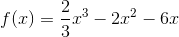.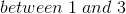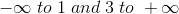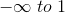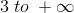Explanation:

We find the region of decreasing of the function by taking the derivative and setting it equal to zero.  Then, we plug numbers to the left and right of the roots into the derivative.  If it is positive, the function is increasing.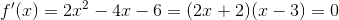.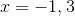If we plug in more negative values, the derivative function is positive.  Therefore, we must be increasing until we get to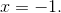In between the roots, the derivative is negative (so, we ignore that).  If we plug values higher than, our derivative function is again positive, so we switch back to increasing after the second root.

### Example Question #42 : Intervals

Find the increasing intervals of the following function: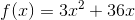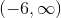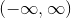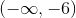The function is never increasingExplanation:

To determine the intervals on which the function is increasing, we must find the intervals on which the first derivative is positive.

The first derivative of the function is equal to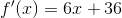and was found using the following rule: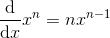Next, we must find the critical values, at which the first derivative is equal to zero: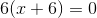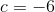Now, using the critical value, we make our intervals: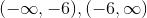Note that at the bounds of the interval, the first derivative is neither positive nor negative.

To determine whether the derivative is positive or negative on each interval, simply plug any value on each interval into the first derivative function; on the first interval, the first derivative is negative, and on the second interval it is positive. Thus, the function is increasing on### Example Question #43 : Intervals

Find the intervals on which the following function is increasing: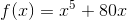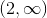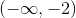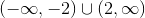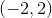Explanation:

To find the intervals on which the function is increasing, we must find the intervals on which the first derivative of the function is positive.

The first derivative of the function is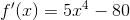and was found using the following rule:Next, we must find the critical values, or values at which the first derivative of the function is equal to zero: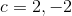Now, using the critical values, we can create the intervals: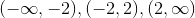Note that at the endpoints of the intervals, the first derivative is neither positive nor negative.

To determine the sign of the first derivative on each interval, simply plug in any value on each interval into the first derivative function and check the sign. On the first interval, the first derivative is positive, on the second it is negative, and on the third it is positive. Thus, the intervals on which the function is increasing are.

### Example Question #44 : Intervals

Tell whether g(x) is increasing or decreasing on the interval [0,3], and how you know.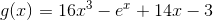The function is decreasing on the interval [0,3], because the first derivative is negative.

The function is increasing on the interval [0,3], because the first derivative is negative.

The function is decreasing on the interval [0,3], because the first derivative is positive.

The function is increasing on the interval [0,3], because the first derivative is positive.

The function is increasing on the interval [0,3], because the first derivative is positive.

Explanation:

Tell whether g(x) is increasing or decreasing on the interval [0,3], and how you know.To tell if a function is increasing or decreasing, we need to see where its derivative is positive and negative. Wherever the derivative is negative, the function will be decreasing, and vice versa.

So, let' find g'(x)

Recall that the derivative of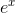is just. Also, any exponent term can be derived by multiplying the term by its exponent and then decreasing the exponent by 1.

So, we get: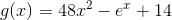Next, plug in the endpoints of our function and see what we get for a sign: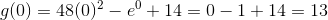Positive, so we are increasing at 0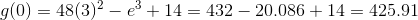So our endpoints both yield a positive derivative. If we tested any point between them, we would also get a positive derivative.

This means that our function is increasing on the interval [0,3], because our first derivative is positive.

### Example Question #45 : Intervals

Is the function g(x) increasing or decreasing on the interval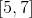?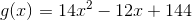Decreasing, because g'(x) is positive on the interval.

Increasing, because g'(x) is negative on the interval.

Increasing, because g'(x) is positive on the interval.

Decreasing, because g'(x) is negative on the interval.

Increasing, because g'(x) is positive on the interval.

Explanation:

Is the function g(x) increasing or decreasing on the interval?To tell if a function is increasing or decreasing, we need to see if its first derivative is positive or negative. let's find g'(x)

Recall that the derivative of a polynomial can be found by taking each term, multiplying by its exponent and then decreasing the exponent by 1.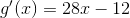Next, we need to see if the function is positive or negative over the given interval.

Begin by finding g'(5)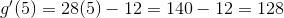So, g'(5) is positive. Furthermore, any number greater than 5 will also be positive. This means our function is increasing on the given interval

Increasing, because g'(x) is positive on the interval.

1 3 Next →